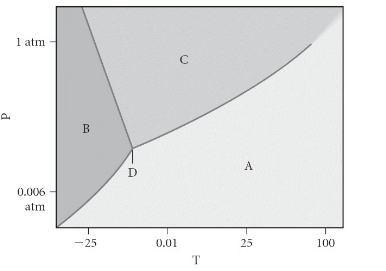# Problem: Choose the answer that correctly assigns the labels on the phase.A) A = liquid, B = gas, C = solid, D = triple pointB) A = gas, B = liquid, C = solid, D = critical pointC) A = liquid, B = solid, C = gas, D = critical pointD) A = gas, B = solid, C = liquid, D = triple pointE) A = solid, B = gas, C = liquid, D = supercritical fluid

###### FREE Expert Solution
100% (458 ratings)
###### FREE Expert Solution

We’re being asked to label the phases on the given phase diagram. Recall that a phase diagram shows the transition of matter between solid, liquid, and gas phases as temperature and pressure changes.

In a phase diagram, the different phases can be identified by their location:

• Solid: can be found at high pressure and low temperature

• Liquid: can be found between the solid and gas regions

• Gas: can be found at low pressure and high temperature

• Triple Point: the point at which all three phases are in equilibrium

• Critical Point: the point at which the liquid and gas phases coexist. The resulting phase is a supercritical fluid.

100% (458 ratings)###### Problem Details

Choose the answer that correctly assigns the labels on the phase.

A) A = liquid, B = gas, C = solid, D = triple point

B) A = gas, B = liquid, C = solid, D = critical point

C) A = liquid, B = solid, C = gas, D = critical point

D) A = gas, B = solid, C = liquid, D = triple point

E) A = solid, B = gas, C = liquid, D = supercritical fluidWhat scientific concept do you need to know in order to solve this problem?

Our tutors have indicated that to solve this problem you will need to apply the Phase Diagram concept. You can view video lessons to learn Phase Diagram. Or if you need more Phase Diagram practice, you can also practice Phase Diagram practice problems.

What is the difficulty of this problem?

Our tutors rated the difficulty ofChoose the answer that correctly assigns the labels on the p...as medium difficulty.

How long does this problem take to solve?

Our expert Chemistry tutor, Sabrina took 1 minute and 53 seconds to solve this problem. You can follow their steps in the video explanation above.

What professor is this problem relevant for?

Based on our data, we think this problem is relevant for Professor Selampinar's class at UCONN.